Show TOC

# AggregationGeneral

Aggregation is the combination or compression of various data, measured values, or indicators to superordinate key figures using certain rules (aggregation rules). It is used for a spatially, temporally, or factually compressed display. In this way, you can aggregate the measured values of a measurement network by calculating their arithmetic average.

In the Central Performance History (CPH), aggregation is used primarily to reduce the memory requirement of the database. Collected performance values are only stored for a defined period of time in the CPH before they are aggregated and the original data is deleted. Inevitably, each aggregation means information is lost; however, without aggregation, the space requirement of the CPH would exceed all limitations in the long term. There are two different methods of aggregation:

• ·        Reducing the Granularity

You can aggregate data by reducing the temporal granularity; that is, for example, calculating the average values for the day from the average values per hour: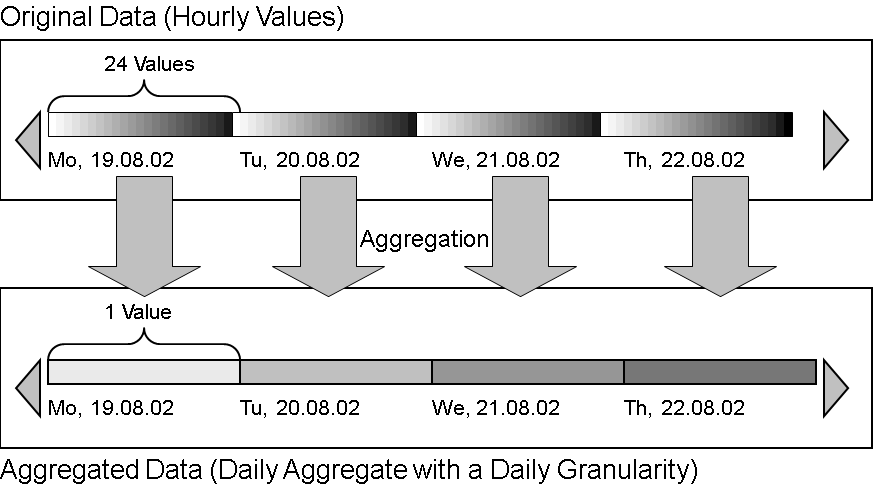Tip

With this form of aggregation, a daily aggregate is calculated from 24 hourly values. This aggregate can also be described as the daily aggregate with a daily granularity (this is expressed in a complicated way, but is useful for differentiating this method from the second method, described below).

• ·        Combination of Analogous Time Periods

The period from which the values are taken to be aggregated into one value does not need to be continuous. This means that you can, for example, also combine the values for a particular hour (such as from 12:00 to 13:00) for all days of a week.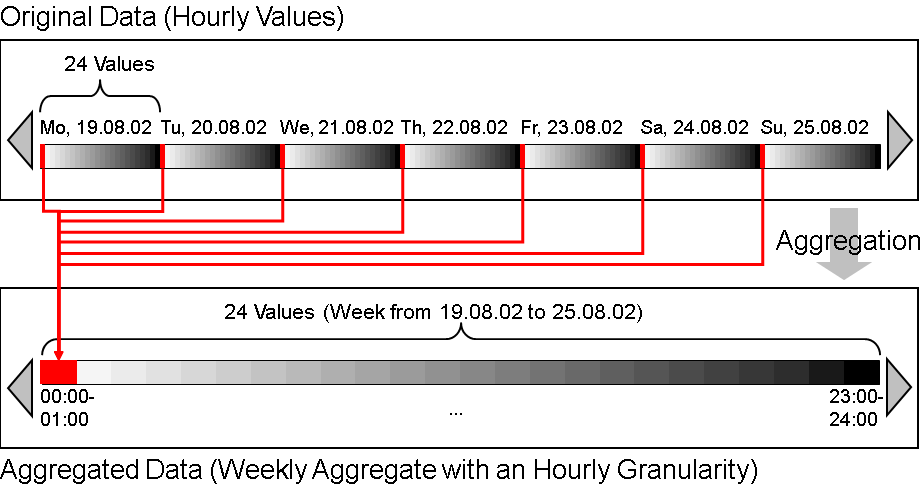Tip

This means that with this form of aggregation, 24 values are calculated from the 7 x 24 hourly values for a week. Each of these 24 new values contains a value for a particular hour of the day for all days of the week. This aggregate is therefore described as a weekly aggregate with an hourly granularity.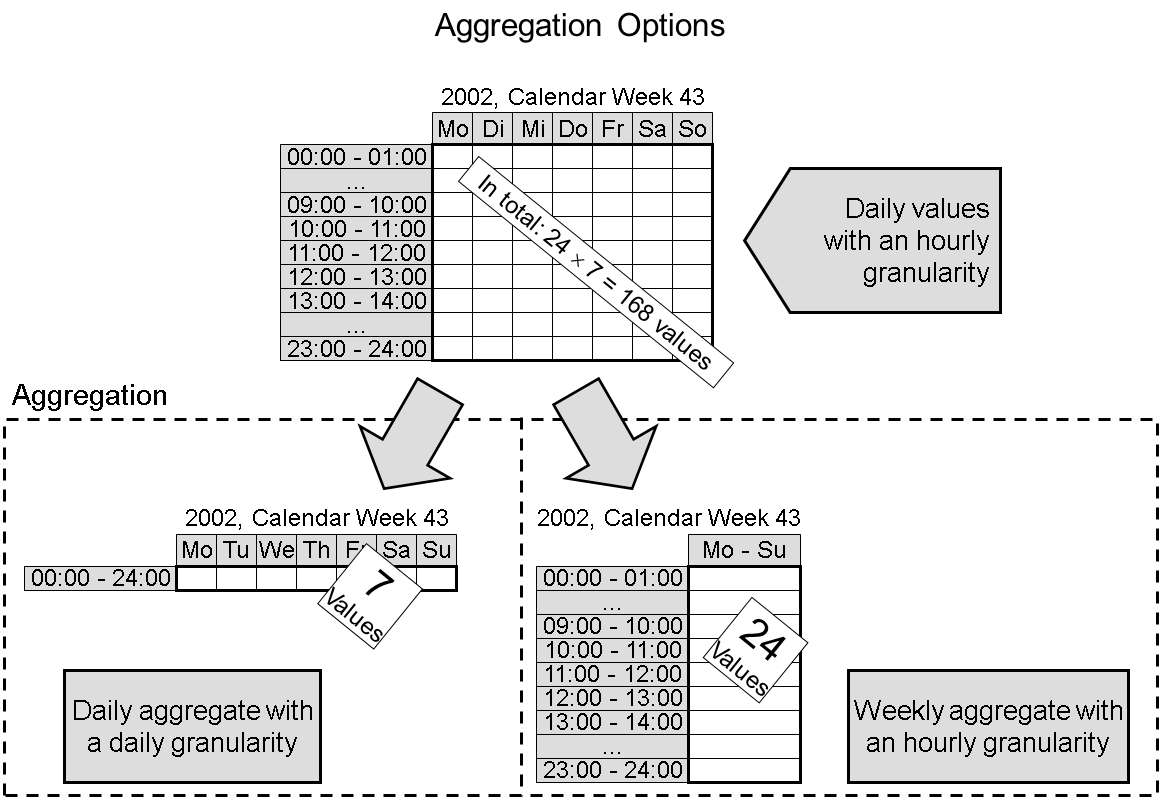Comparison of the Methods
• ·        Reducing the Granularity

This method is particularly suitable for investigate changes of performance attributes over time; for example, for analyzing response times in the case of increasing workload. As a value always relates to a concrete, continuous time period (an hour, a day, a month, and so on), you can identify tendencies more easily with this type of aggregation by comparing the development of values for time periods of this type that follow on from each other.

• ·        Combination of Analogous Time Periods

With this method, you simulate the higher temporal granularity of the data before aggregation, but combine time periods in which similar values are expected. In this way, you could expect that in a stably functioning system with constant total workload, the performance values would be roughly the same every Monday between 12:00 and 13:00. You can therefore identify cyclical changes (such as during the course of the day) more easily, but gradual, continuous changes less easily.

For the identification of cyclical changes, such as workload spikes repeated daily, this type of aggregation even has the advantage over the original data that the aggregation functions like an extension of a random test, and therefore minimizes coincidental variations.

Note

The two types of aggregation described are not mutually exclusive. If necessary, you can use both methods in parallel to aggregate the collected performance values.

Example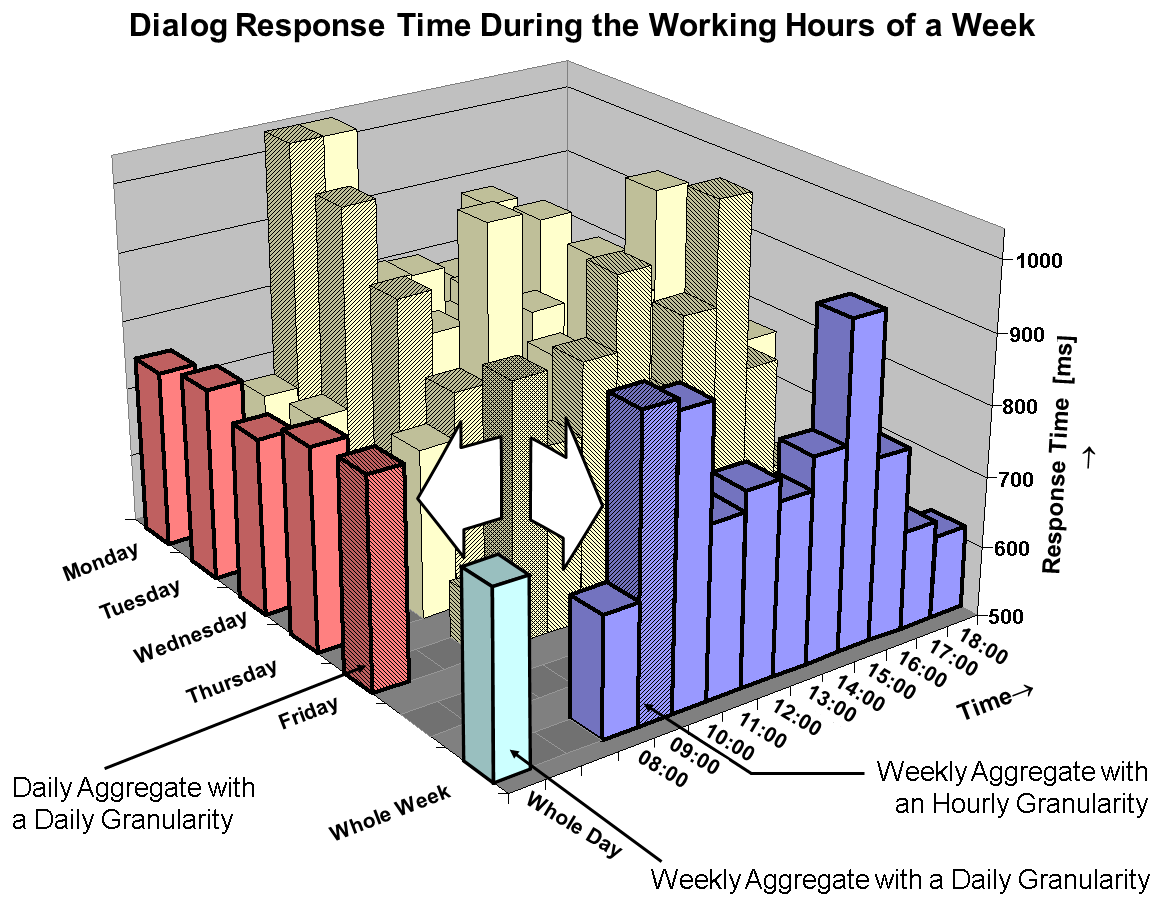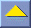Central Performance History of the Monitoring Architecture start page